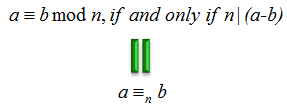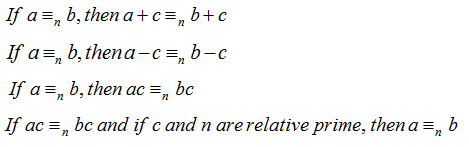Engineering Math - Quick Reference                                 Home : www.sharetechnote.com Modulo Math : Modular Equivalence   Two integers are modular equivalent (equivalent mod n) if they gives the same remainder when it is divided by n.   Example 01 > Two integer 2 and 7 are (equivalent mod 5) because (2 mod 5) = (7 mod 5) Two integer 2 and 12 are (equivalent mod 5) because (2 mod 5) = (12 mod 5) Two integer 2 and 17 are (equivalent mod 5) because (2 mod 5) = (17 mod 5)   Formal Definition   If you are scared of mathematical symbol, forget about this and just stick to the definition explained above. You can come back later when you become more familiar/comfortable to mathematical symbols. Anyway, the formal expression of the definition of Modular Equivalence is as follows :Properties of Modulo Equivalence.   Modulo Equivalence has following properties. Don't try to memorize this. Just try to understand what does it mean by each of the properties for now. When you see some real application that would use some of these properties and you can come back here and you will underand the practical importance of these properties.